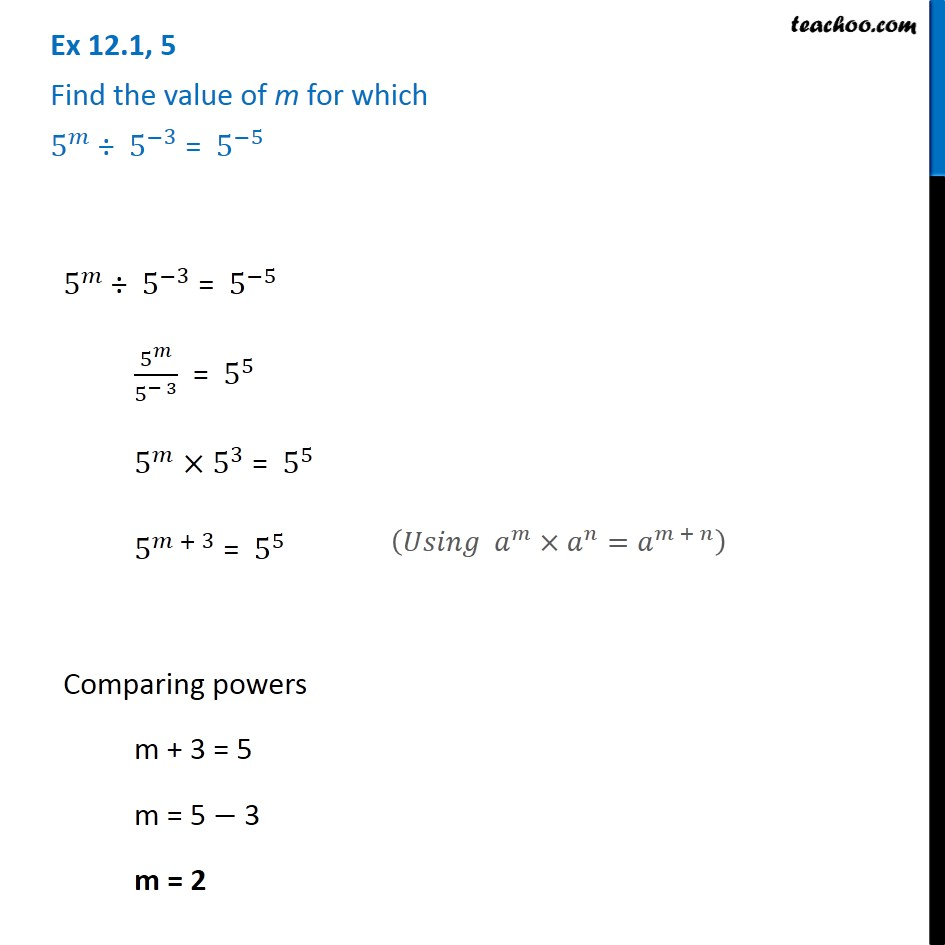Ex 12.1

Chapter 12 Class 8 Exponents and Powers
Serial order wise641 students have Teachoo Black. What are you waiting for?

### Transcript

Ex 12.1, 5 Find the value of m for which 5^𝑚 ÷ 5^(−3) = 5^(−5) 5^𝑚 ÷ 5^(−3) = 5^(−5) 5^𝑚/5^(− 3) = 5^5 5^𝑚×5^3 = 5^5 5^(𝑚 + 3) = 5^5 Comparing powers m + 3 = 5 m = 5 − 3 m = 2 (𝑈𝑠𝑖𝑛𝑔 𝑎^𝑚×𝑎^𝑛=𝑎^(𝑚 + 𝑛) )